# Converting Repeating Decimals to Fractions Lesson Plan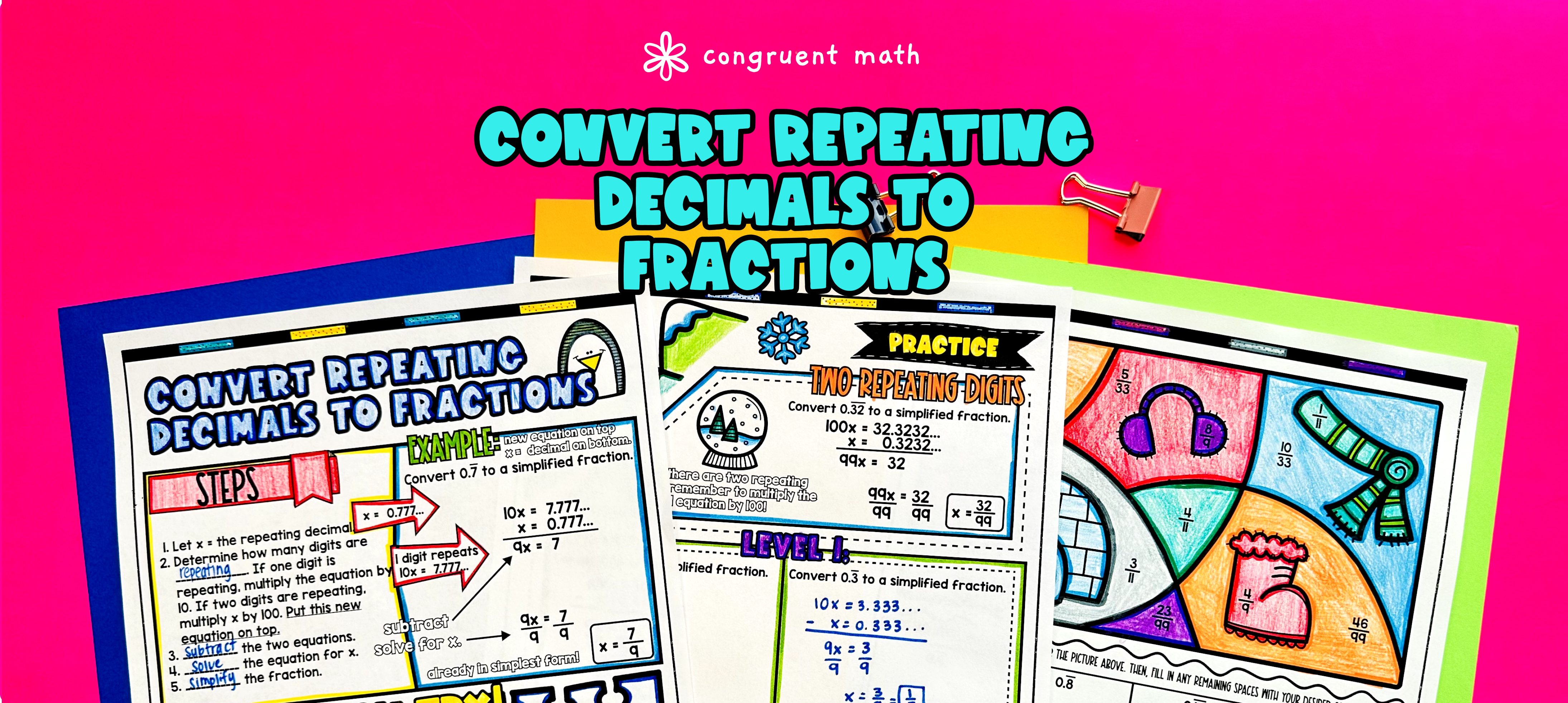Pin This

## Overview

Ever wondered how to teach converting repeating decimals to fractions in an engaging way to your eighth-grade students?

In this lesson plan, students will learn about converting from repeating decimals to fractions and their real-life applications. Through artistic, interactive guided notes, checks for understanding, a practice color by number activity, and a maze worksheet, students will gain a comprehensive understanding of converting repeating decimals to fractions.

The lesson culminates with a real-life example that explores how rational number conversions are used in practical situations. Students will read and write about the real-life applications of converting repeating decimals to fractions.

## Get the Lesson Materials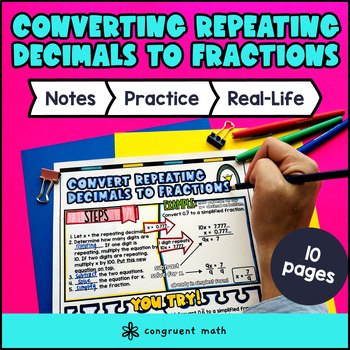\$4.25

## Learning Objectives

After this lesson, students will be able to:

• Convert repeating decimals to fractions (decimals with 1 or 2 repeating digits)

• Explain the real-life applications of converting repeating decimals to fractions

## Prerequisites

Before this lesson, students should be familiar with:

• Basic understanding of equations, variables

• How to solve one step equations

## Key Vocabulary

• Repeating decimal

• Fraction

• Rational number

## Procedure

### Introduction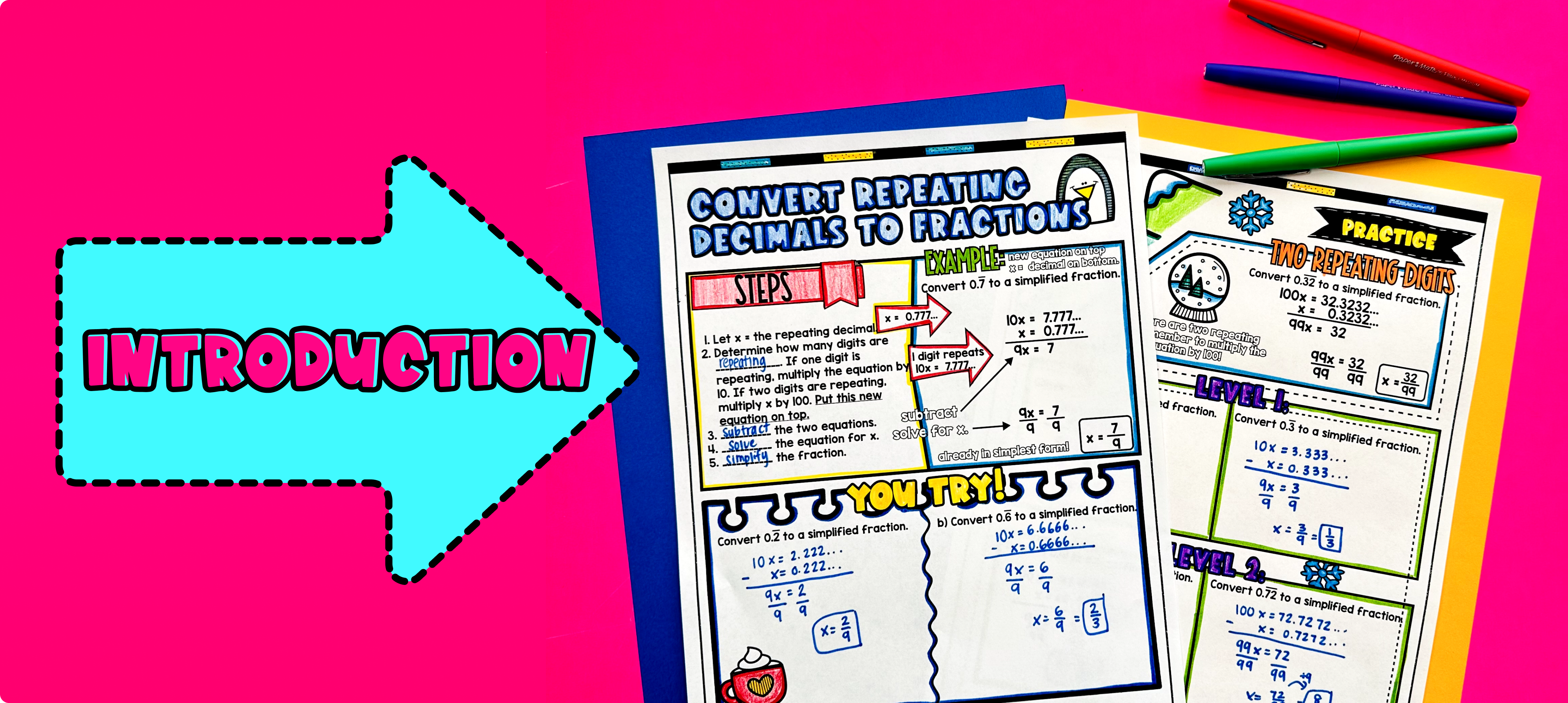Pin This

As a hook, ask students why converting repeating decimals to fractions is important in real-life situations. Refer to the last page of the guided notes for ideas.

Use the first page of the guided notes to introduce the concept of converting repeating decimals to fractions. Walk through the key points of the topic, including the notation used to represent repeating decimals, the process of converting repeating decimals to fractions, and how to set up and solve the equations. Refer to the guided notes for examples and practice.

Based on student responses, reteach concepts that students need extra help with. If your class has a wide range of proficiency levels, you can pull out students for reteaching, and have more advanced students begin work on the practice exercises.

### Practice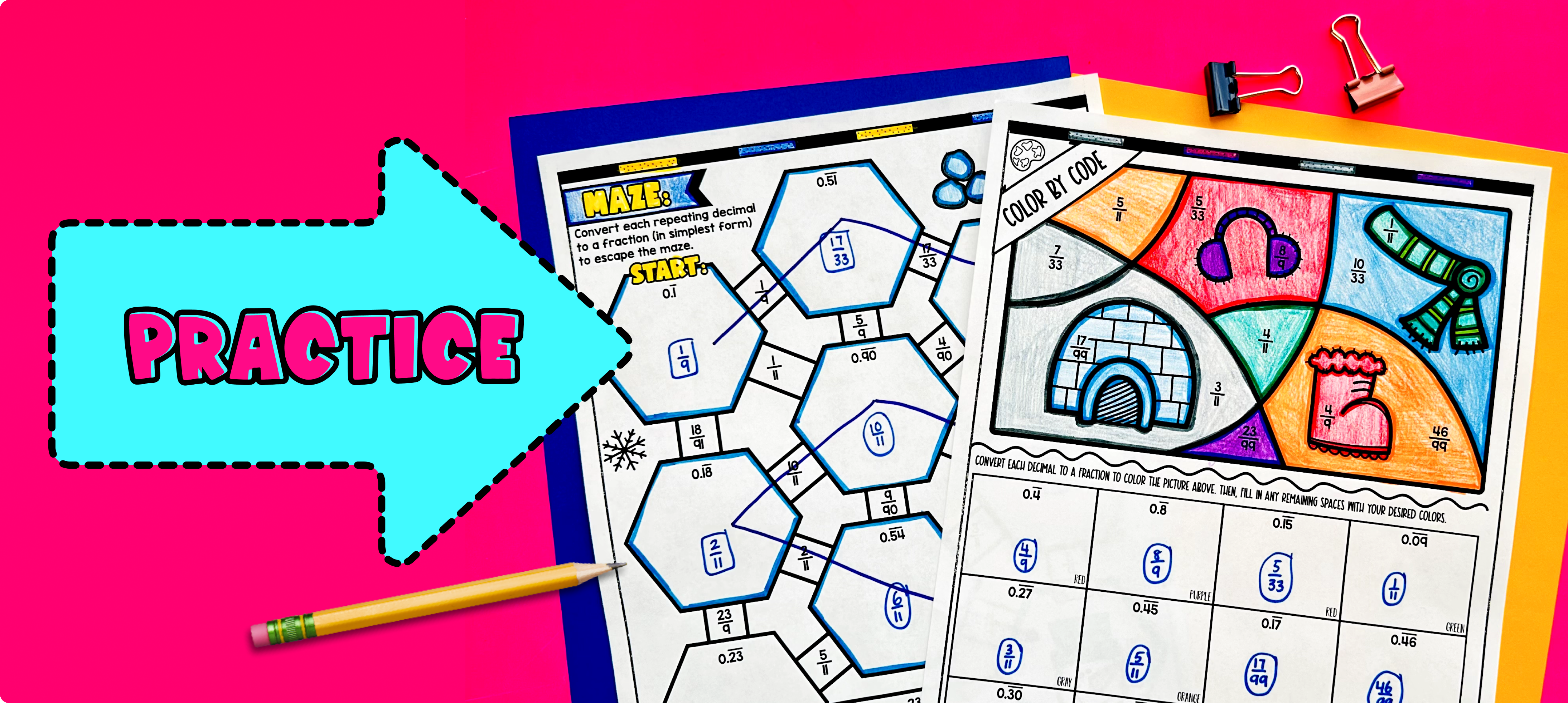Pin This

Have students practice converting from repeating decimals (one or two repeating digits) to fractions using the practice worksheet on page 2 of the guided notes. Walk around to answer student questions.

Fast finishers can dive into the maze or color by number activity for extra practice. You can assign it as homework for the remainder of the class.

### Real-Life Application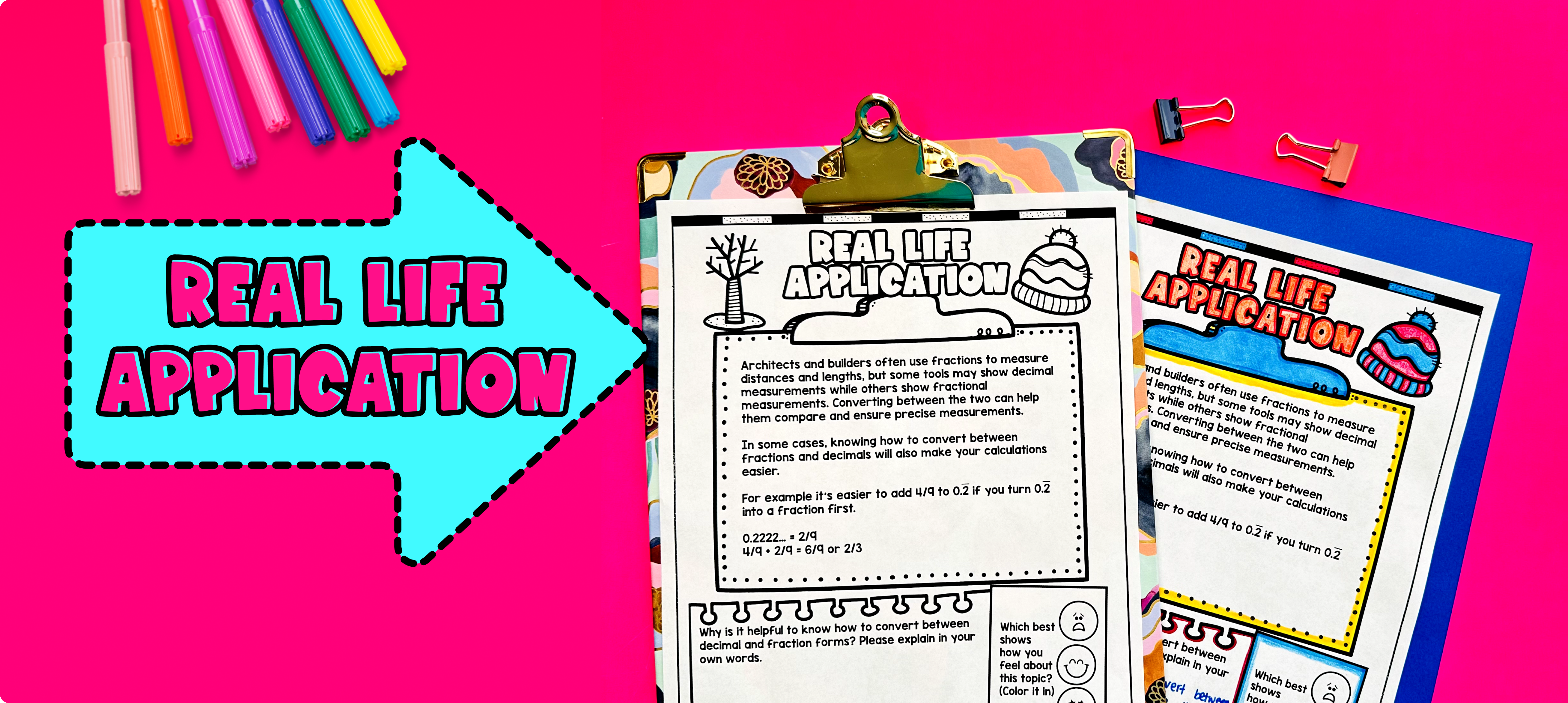Pin This

Bring the class back together, and introduce the concept of real-life applications for converting repeating decimals to fractions. Explain that understanding how to convert repeating decimals to fractions can be useful in various real-life situations that involve measurements, calculations, and comparisons.

Refer to the last page of the guided notes for more ideas on how to teach the real-life applications of converting repeating decimals to fractions.